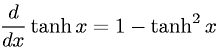Equations > Calculus > Differentiation/Differentals > Derivative of Hyperbolic Tangent

### Derivative of Hyperbolic TangentLatex Code:

MathML Code:

 $\fracd\mathrm{dx}\mathrm{tanh}x=1-{\mathrm{tanh}}^{2}x$

MathType 5.0: